# AP SSC Board Question Paper for Class 10th Science Paper 1 2018 in PDF

## AP Board 10th Standard Science Paper 1 Exam Question Papers 2018 with Solutions – Free Download

The AP SSC (Andhra Pradesh board) Class 10 Question Paper Science Paper 1 2018 with solutions are used by the students to prepare most competently for the exams. This Class 10 exam question paper brings solutions that are crafted after proper research and will help students to ace the Science subject. Students can find the downloadable PDF formats of these solved or the unsolved question papers, as required, from the respective links. Students can also refer to the solutions and analyse their progress in exam preparations. In the article below, the clickable links to download the papers are mentioned.

Science is a very interesting subject and scoring high marks in it, requires hard work and commitment. Students will have to master the entire concepts of the subject, if they want to crack the subject. Performing well in the AP Board Class 10 Science exam is easy, if a student knows the complex formulas and equations of Class 10 Science, thoroughly. For this, students are advised to practise the previous year papers of AP Board Class 10 Science, regularly. This will also provide an insight on the question paper design, marking scheme, exam pattern and more. These solutions are the best resource for a student to gauge their preparation and the difficulty level of the questions that are mostly asked in the board exams.

### AP Board Class 10 Science Paper 1 2018 Question Paper with Solutions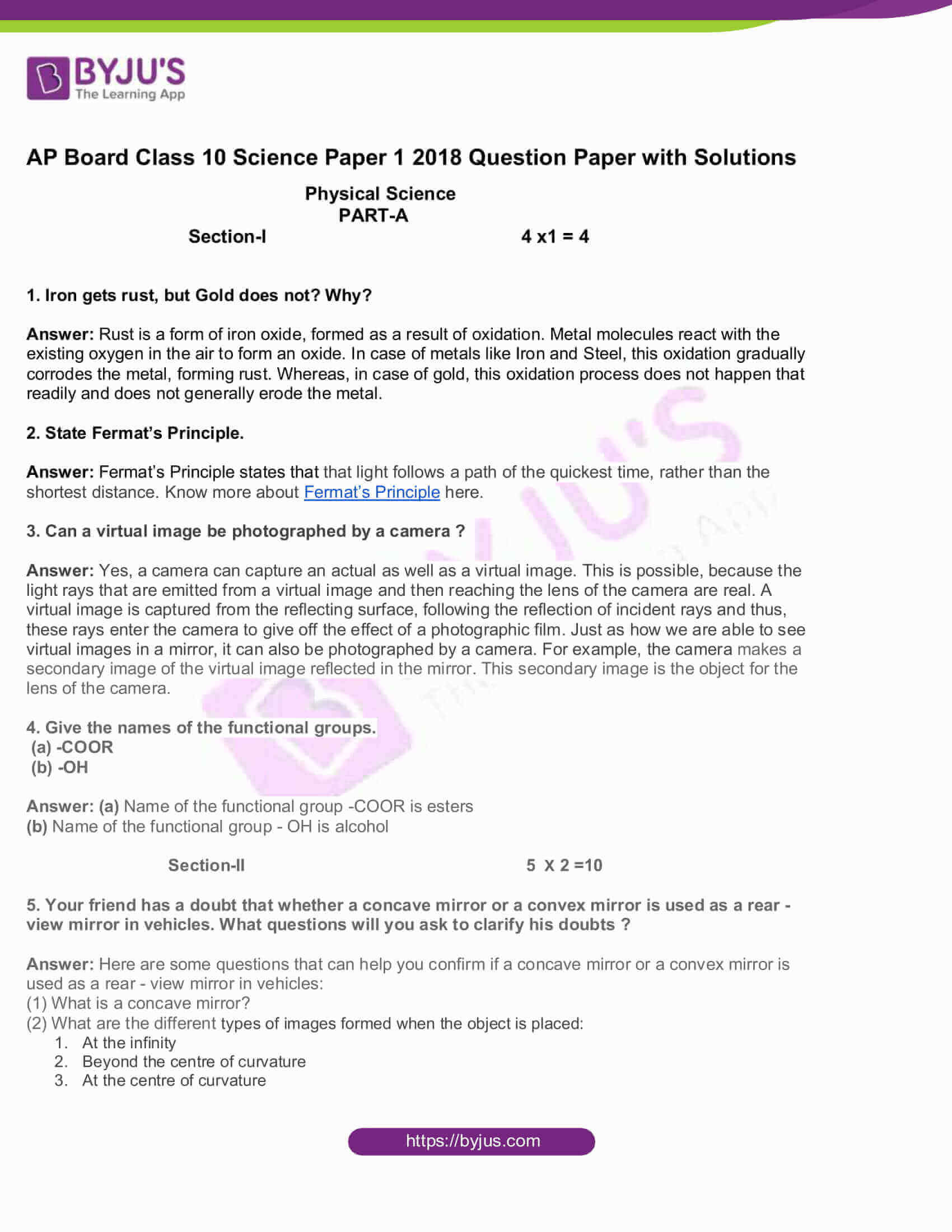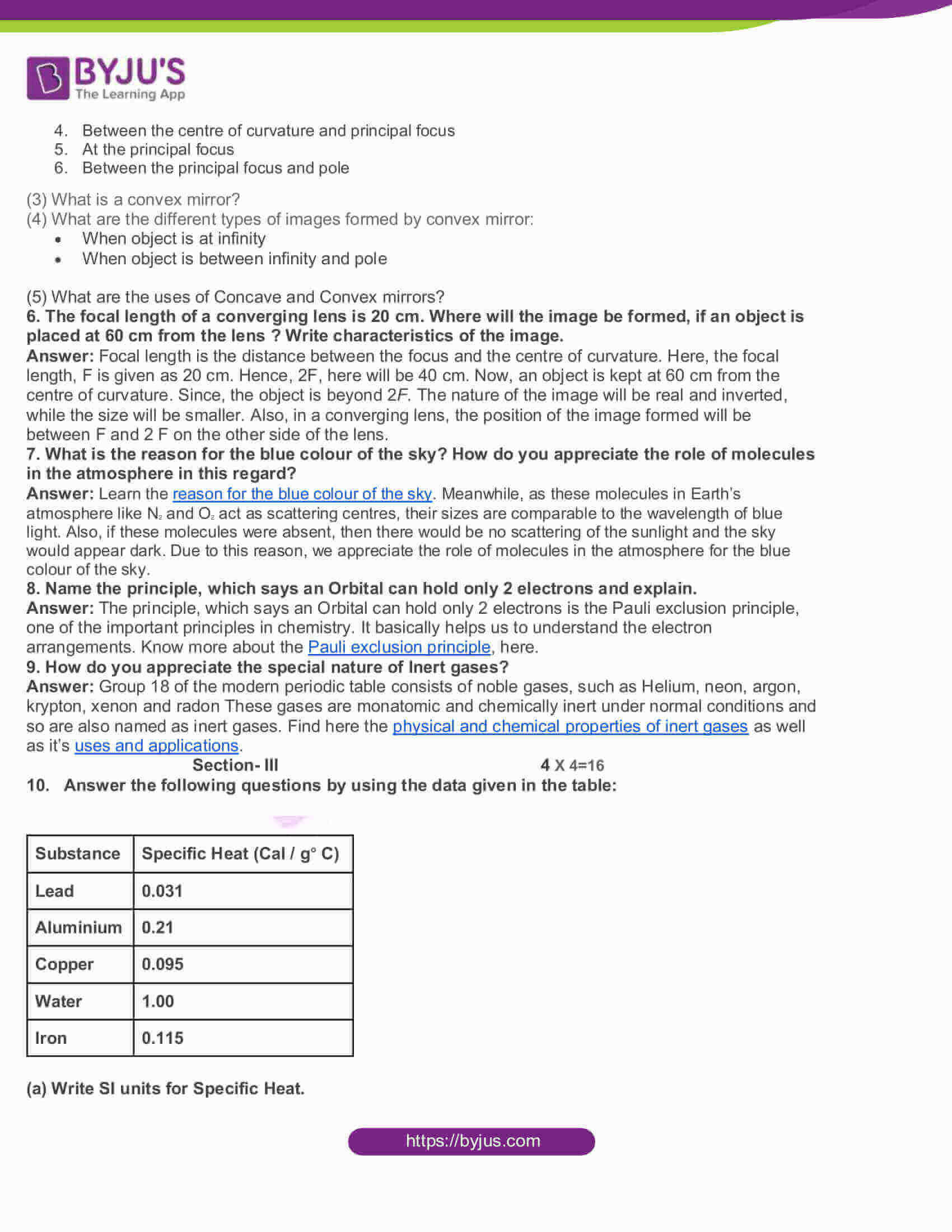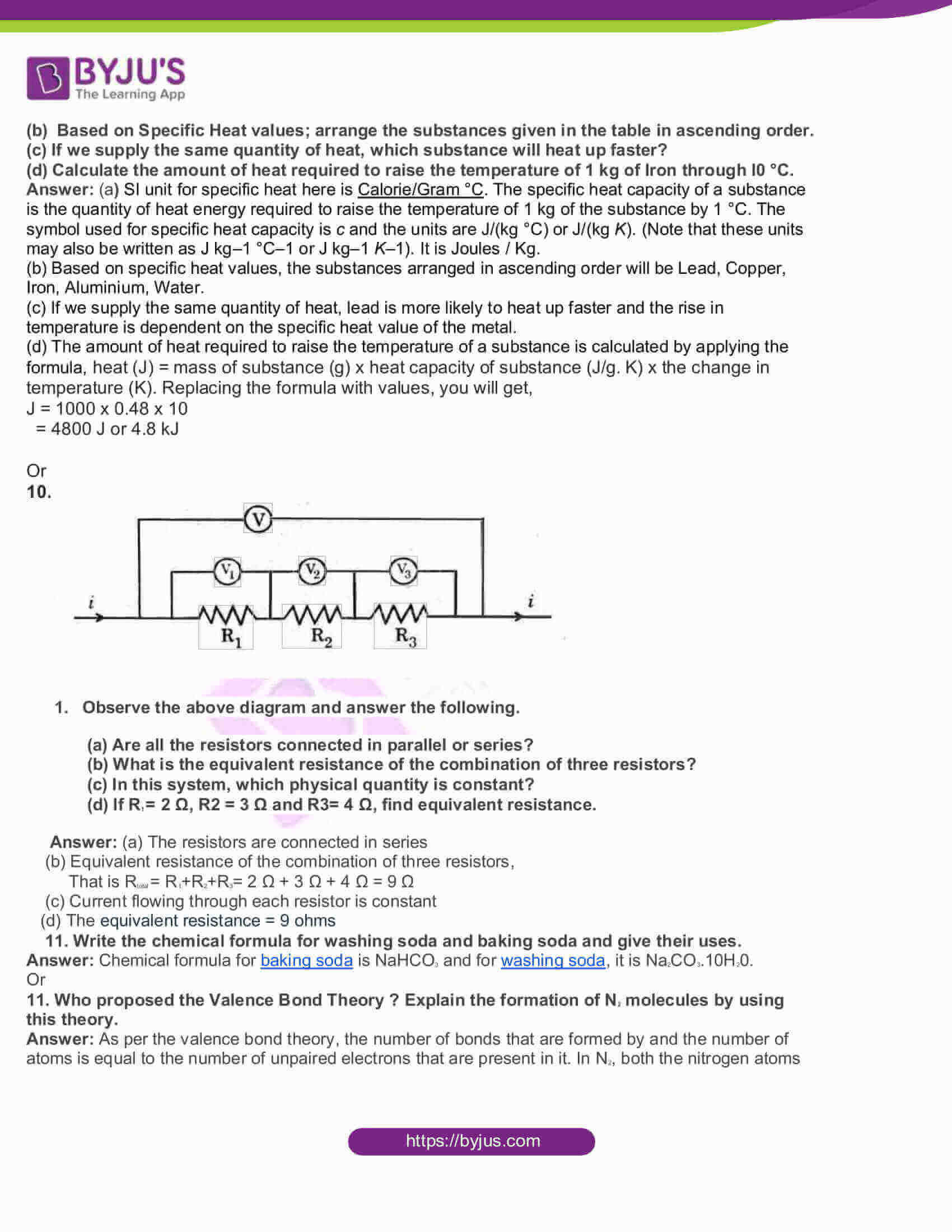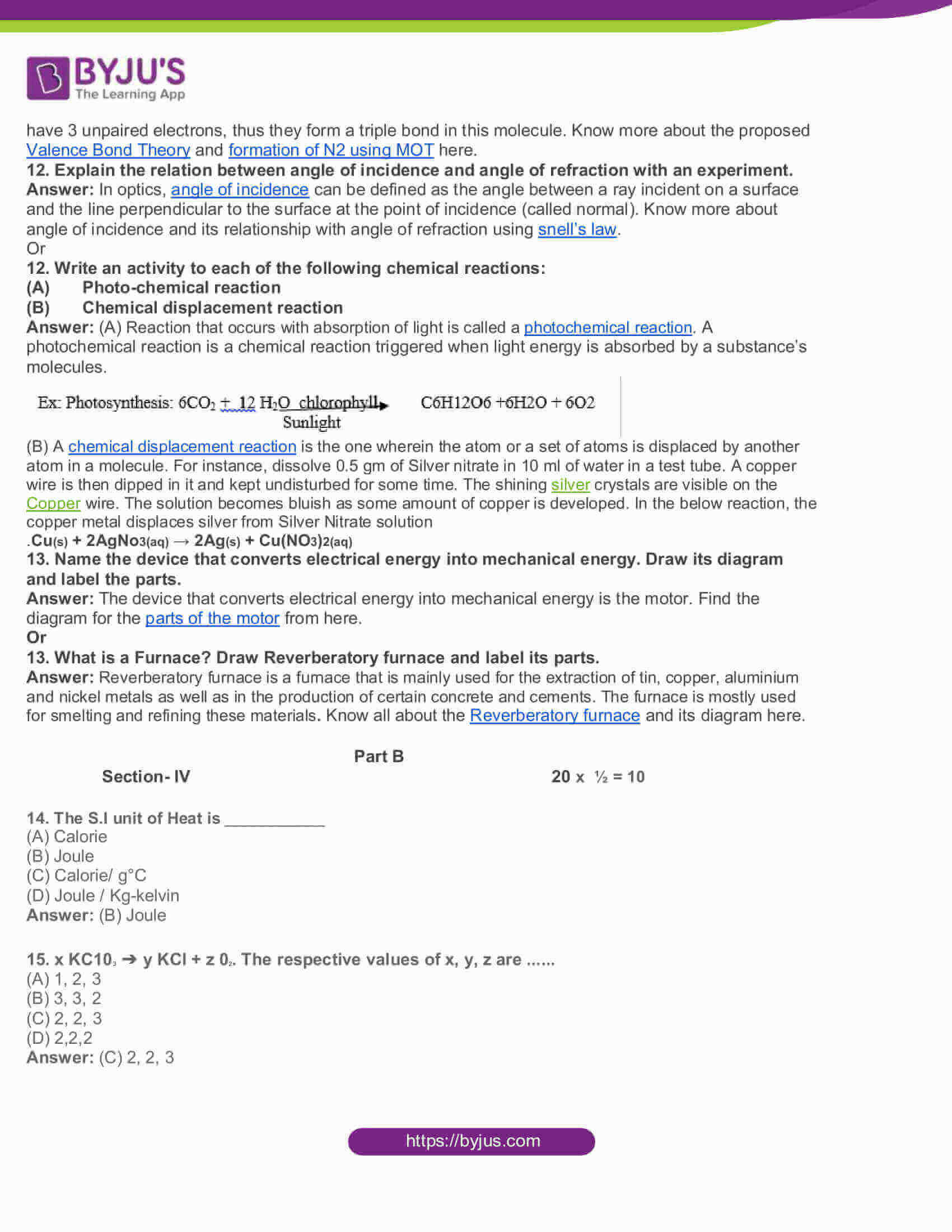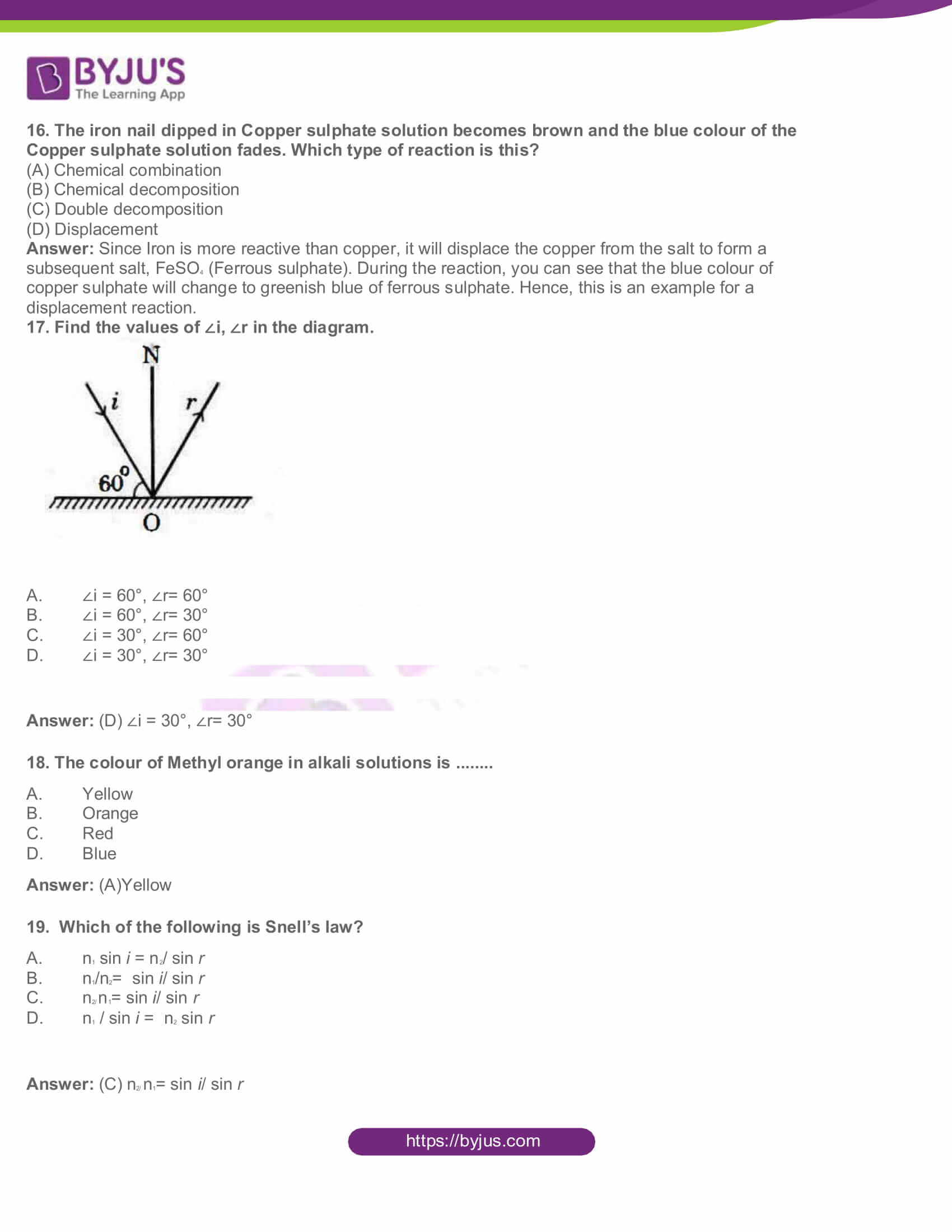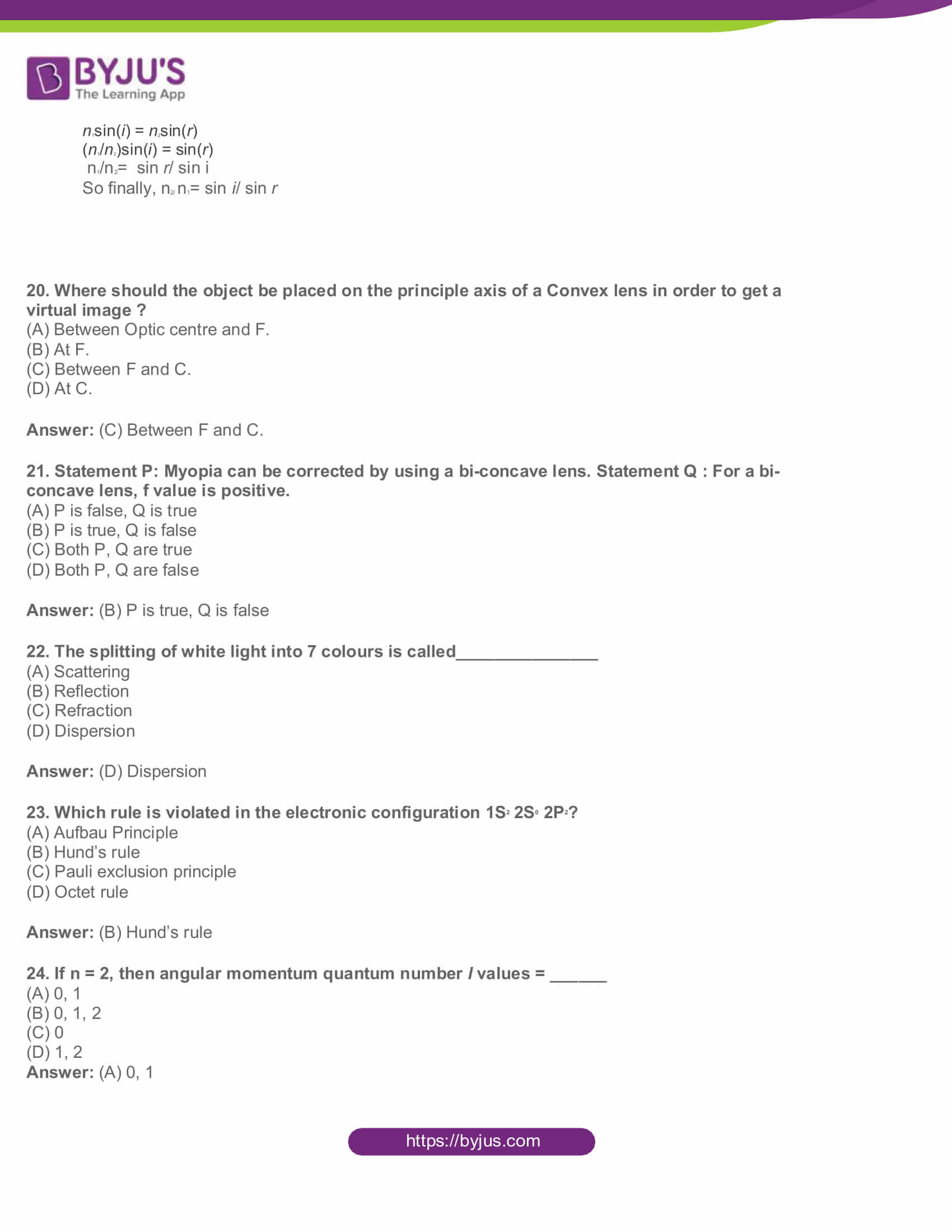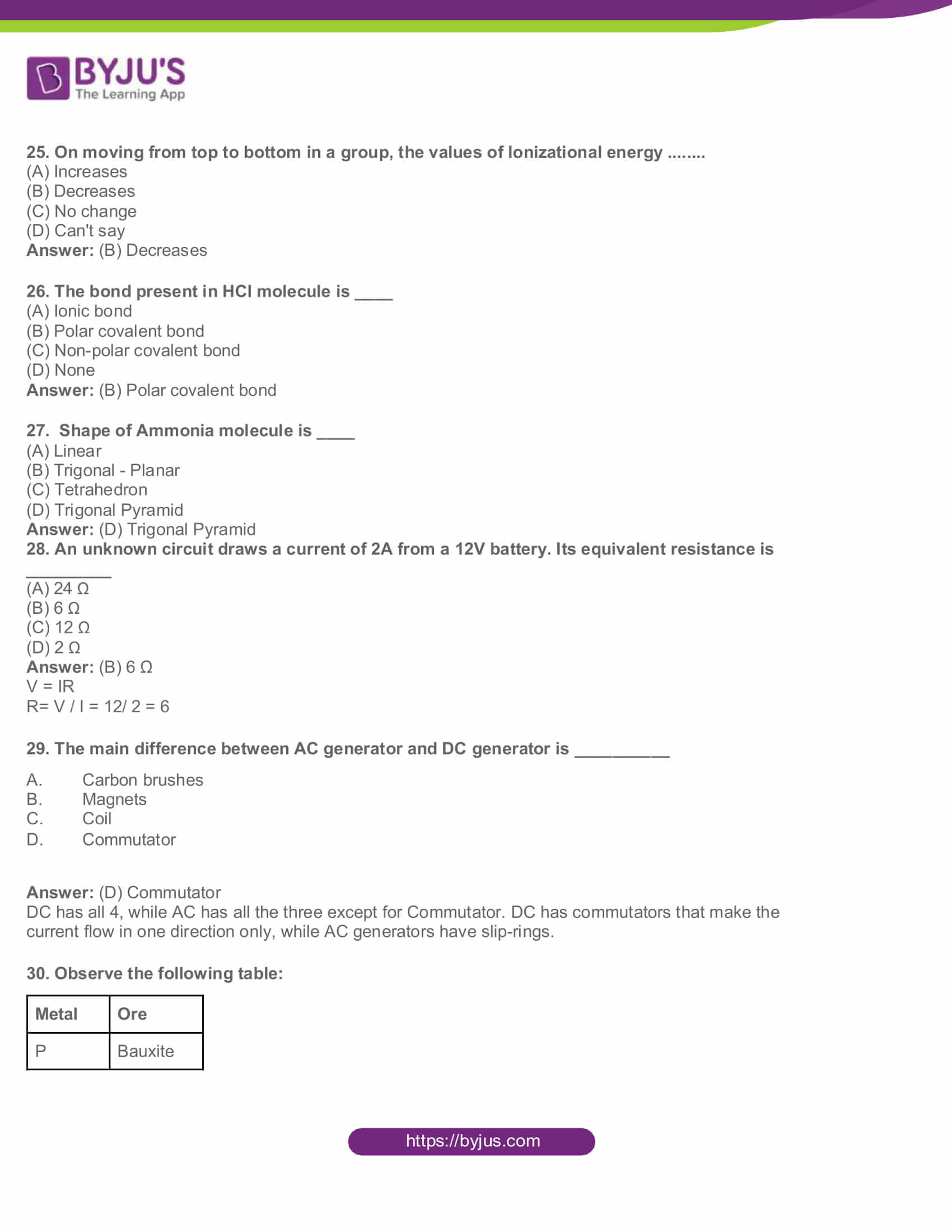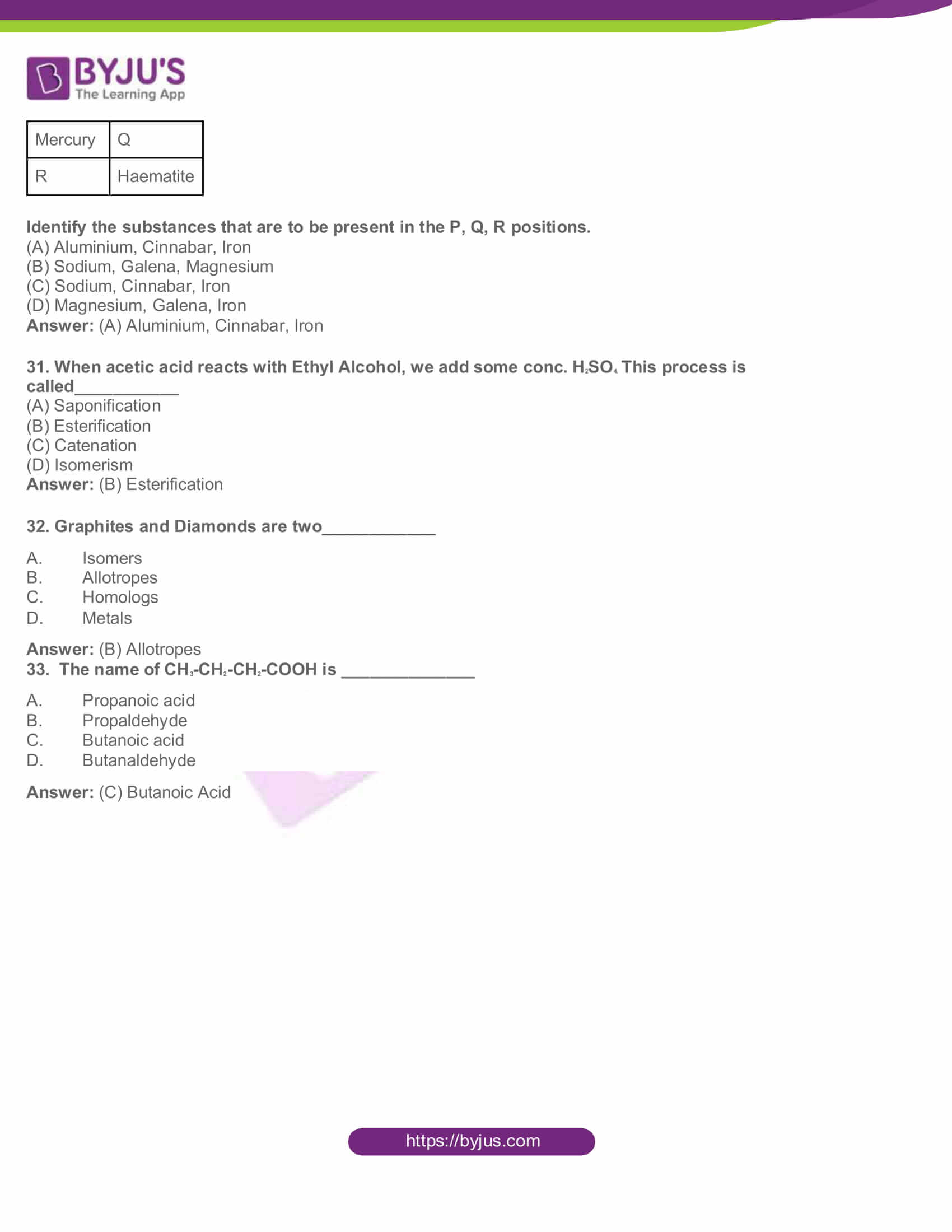Physical Science

PART-A

Section-I 4 x1 = 4

1. Iron gets rust, but Gold does not? Why?

Answer: Rust is a form of iron oxide, formed as a result of oxidation. Metal molecules react with the existing oxygen in the air to form an oxide. In case of metals like Iron and Steel, this oxidation gradually corrodes the metal, forming rust. Whereas, in case of gold, this oxidation process does not happen that readily and does not generally erode the metal.

2. State Fermat’s Principle.

Answer: Fermat’s Principle states that that light follows a path of the quickest time, rather than the shortest distance. Know more about Fermat’s Principle here.

3. Can a virtual image be photographed by a camera ?

Answer: Yes, a camera can capture an actual as well as a virtual image. This is possible, because the light rays that are emitted from a virtual image and then reaching the lens of the camera are real. A virtual image is captured from the reflecting surface, following the reflection of incident rays and thus, these rays enter the camera to give off the effect of a photographic film. Just as how we are able to see virtual images in a mirror, it can also be photographed by a camera. For example, the camera makes a secondary image of the virtual image reflected in the mirror. This secondary image is the object for the lens of the camera.

4. Give the names of the functional groups.

(a) -COOR

(b) -OH

Answer: (a) Name of the functional group -COOR is esters

(b) Name of the functional group – OH is alcohol

Section-II 5 X 2 =10

5. Your friend has a doubt that whether a concave mirror or a convex mirror is used as a rear – view mirror in vehicles. What questions will you ask to clarify his doubts ?

Answer: Here are some questions that can help you confirm if a concave mirror or a convex mirror is used as a rear – view mirror in vehicles:

(1) What is a concave mirror?

(2) What are the different types of images formed when the object is placed:

1. At the infinity
2. Beyond the centre of curvature
3. At the centre of curvature
4. Between the centre of curvature and principal focus
5. At the principal focus
6. Between the principal focus and pole

(3) What is a convex mirror?

(4) What are the different types of images formed by convex mirror:

• When object is at infinity
• When object is between infinity and pole

(5) What are the uses of Concave and Convex mirrors?

6. The focal length of a converging lens is 20 cm. Where will the image be formed, if an object is placed at 60 cm from the lens ? Write characteristics of the image.

Answer: Focal length is the distance between the focus and the centre of curvature. Here, the focal length, F is given as 20 cm. Hence, 2F, here will be 40 cm. Now, an object is kept at 60 cm from the centre of curvature. Since, the object is beyond 2F. The nature of the image will be real and inverted, while the size will be smaller. Also, in a converging lens, the position of the image formed will be between F and 2 F on the other side of the lens.

7. What is the reason for the blue colour of the sky? How do you appreciate the role of molecules in the atmosphere in this regard?

Answer: Learn the reason for the blue colour of the sky. Meanwhile, as these molecules in Earth’s atmosphere like N2 and O2 act as scattering centres, their sizes are comparable to the wavelength of blue light. Also, if these molecules were absent, then there would be no scattering of the sunlight and the sky would appear dark. Due to this reason, we appreciate the role of molecules in the atmosphere for the blue colour of the sky.

8. Name the principle, which says an Orbital can hold only 2 electrons and explain.

Answer: The principle, which says an Orbital can hold only 2 electrons is the Pauli exclusion principle, one of the important principles in chemistry. It basically helps us to understand the electron arrangements. Know more about the Pauli exclusion principle, here.

9. How do you appreciate the special nature of Inert gases?

Answer: Group 18 of the modern periodic table consists of noble gases, such as Helium, neon, argon, krypton, xenon and radon These gases are monatomic and chemically inert under normal conditions and so are also named as inert gases. Find here the physical and chemical properties of inert gases as well as it’s uses and applications.

Section- III 4 X 4=16

10. Answer the following questions by using the data given in the table:

 Substance Specific Heat (Cal / g° C) Lead 0.031 Aluminium 0.21 Copper 0.095 Water 1.00 Iron 0.115

(a) Write SI units for Specific Heat.

(b) Based on Specific Heat values; arrange the substances given in the table in ascending order.

(c) If we supply the same quantity of heat, which substance will heat up faster?

(d) Calculate the amount of heat required to raise the temperature of 1 kg of Iron through l0 °C.

Answer: (a) SI unit for specific heat here is Calorie/Gram °C. The specific heat capacity of a substance is the quantity of heat energy required to raise the temperature of 1 kg of the substance by 1 °C. The symbol used for specific heat capacity is c and the units are J/(kg °C) or J/(kg K). (Note that these units may also be written as J kg–1 °C–1 or J kg–1 K–1). It is Joules / Kg.

(b) Based on specific heat values, the substances arranged in ascending order will be Lead, Copper, Iron, Aluminium, Water.

(c) If we supply the same quantity of heat, lead is more likely to heat up faster and the rise in temperature is dependent on the specific heat value of the metal.

(d) The amount of heat required to raise the temperature of a substance is calculated by applying the formula, heat (J) = mass of substance (g) x heat capacity of substance (J/g. K) x the change in temperature (K). Replacing the formula with values, you will get,

J = 1000 x 0.48 x 10

= 4800 J or 4.8 kJ

Or

10.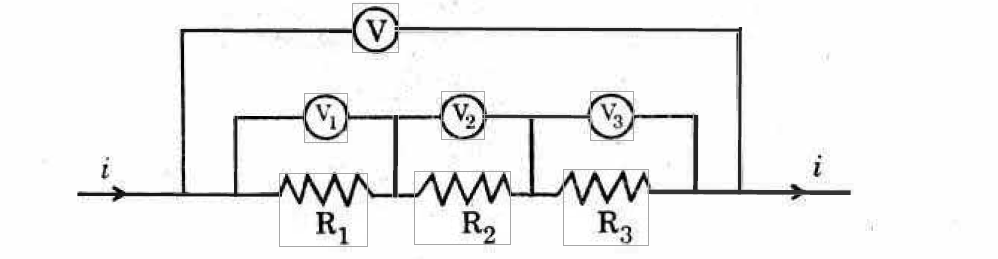1. Observe the above diagram and answer the following.

(a) Are all the resistors connected in parallel or series?

(b) What is the equivalent resistance of the combination of three resistors?

(c) In this system, which physical quantity is constant?

(d) If R1 = 2 Ω, R2 = 3 Ω and R3= 4 Ω, find equivalent resistance.

Answer: (a) The resistors are connected in series

(b) Equivalent resistance of the combination of three resistors,

That is Rtotal = R1+R2+R3= 2 Ω + 3 Ω + 4 Ω = 9 Ω

(c) Current flowing through each resistor is constant

(d) The equivalent resistance = 9 ohms

11. Write the chemical formula for washing soda and baking soda and give their uses.

Answer: Chemical formula for baking soda is NaHCO3 and for washing soda, it is Na2CO3.10H20.

Or

11. Who proposed the Valence Bond Theory ? Explain the formation of N2 molecules by using this theory.

Answer: As per the valence bond theory, the number of bonds that are formed by and the number of atoms is equal to the number of unpaired electrons that are present in it. In N2, both the nitrogen atoms have 3 unpaired electrons, thus they form a triple bond in this molecule. Know more about the proposed Valence Bond Theory and formation of N2 using MOT here.

12. Explain the relation between angle of incidence and angle of refraction with an experiment.

Answer: In optics, angle of incidence can be defined as the angle between a ray incident on a surface and the line perpendicular to the surface at the point of incidence (called normal). Know more about angle of incidence and its relationship with angle of refraction using snell’s law.

Or

12. Write an activity to each of the following chemical reactions:

(A) Photo-chemical reaction

(B) Chemical displacement reaction

Answer: (A) Reaction that occurs with absorption of light is called a photochemical reaction. A photochemical reaction is a chemical reaction triggered when light energy is absorbed by a substance’s molecules.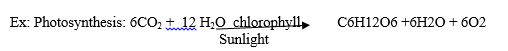(B) A chemical displacement reaction is the one wherein the atom or a set of atoms is displaced by another atom in a molecule. For instance, dissolve 0.5 gm of Silver nitrate in 10 ml of water in a test tube. A copper wire is then dipped in it and kept undisturbed for some time. The shining silver crystals are visible on the Copper wire. The solution becomes bluish as some amount of copper is developed. In the below reaction, the copper metal displaces silver from Silver Nitrate solution

.Cu(s) + 2AgNo3(aq) → 2Ag(s) + Cu(NO3)2(aq)

13. Name the device that converts electrical energy into mechanical energy. Draw its diagram and label the parts.

Answer: The device that converts electrical energy into mechanical energy is the motor. Find the diagram for the parts of the motor from here.

Or

13. What is a Furnace? Draw Reverberatory furnace and label its parts.

Answer: Reverberatory furnace is a furnace that is mainly used for the extraction of tin, copper, aluminium and nickel metals as well as in the production of certain concrete and cements. The furnace is mostly used for smelting and refining these materials. Know all about the Reverberatory furnace and its diagram here.

Part B

Section- IV 20 x ½ = 10

14. The S.I unit of Heat is ___________

(A) Calorie

(B) Joule

(C) Calorie/ g°C

(D) Joule / Kg-kelvin

15. x KC103 ➔ y KCl + z 02. The respective values of x, y, z are ……

(A) 1, 2, 3

(B) 3, 3, 2

(C) 2, 2, 3

(D) 2,2,2

16. The iron nail dipped in Copper sulphate solution becomes brown and the blue colour of the Copper sulphate solution fades. Which type of reaction is this?

(A) Chemical combination

(B) Chemical decomposition

(C) Double decomposition

(D) Displacement

Answer: Since Iron is more reactive than copper, it will displace the copper from the salt to form a subsequent salt, FeSO4 (Ferrous sulphate). During the reaction, you can see that the blue colour of copper sulphate will change to greenish blue of ferrous sulphate. Hence, this is an example for a displacement reaction.

17. Find the values of ∠i, ∠r in the diagram.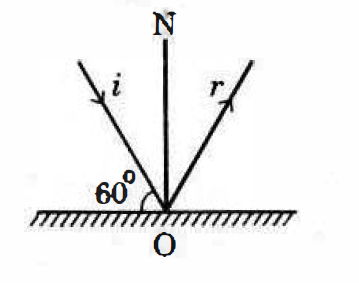1. ∠i = 60°, ∠r= 60°
2. ∠i = 60°, ∠r= 30°
3. ∠i = 30°, ∠r= 60°
4. ∠i = 30°, ∠r= 30°

Answer: (D) ∠i = 30°, ∠r= 30°

18. The colour of Methyl orange in alkali solutions is ……..

1. Yellow
2. Orange
3. Red
4. Blue

19. Which of the following is Snell’s law?

1. n1 sin i = n2/ sin r
2. n1/n2= sin i/ sin r
3. n2/ n1= sin i/ sin r
4. n1 / sin i = n2 sin r

Answer: (C) n2/ n1= sin i/ sin r

n1sin(i) = n2sin(r)

(n1/n2)sin(i) = sin(r)

n1/n2= sin r/ sin i

So finally, n2/ n1= sin i/ sin r

20. Where should the object be placed on the principle axis of a Convex lens in order to get a virtual image ?

(A) Between Optic centre and F.

(B) At F.

(C) Between F and C.

(D) At C.

Answer: (C) Between F and C.

21. Statement P: Myopia can be corrected by using a bi-concave lens. Statement Q : For a bi-concave lens, f value is positive.

(A) P is false, Q is true

(B) P is true, Q is false

(C) Both P, Q are true

(D) Both P, Q are false

Answer: (B) P is true, Q is false

22. The splitting of white light into 7 colours is called_______________

(A) Scattering

(B) Reflection

(C) Refraction

(D) Dispersion

23. Which rule is violated in the electronic configuration 1S2 2S0 2P2?

(A) Aufbau Principle

(B) Hund’s rule

(C) Pauli exclusion principle

(D) Octet rule

24. If n = 2, then angular momentum quantum number l values = ______

(A) 0, 1

(B) 0, 1, 2

(C) 0

(D) 1, 2

25. On moving from top to bottom in a group, the values of Ionizational energy ……..

(A) Increases

(B) Decreases

(C) No change

(D) Can’t say

26. The bond present in HCl molecule is ____

(A) Ionic bond

(B) Polar covalent bond

(C) Non-polar covalent bond

(D) None

27. Shape of Ammonia molecule is ____

(A) Linear

(B) Trigonal – Planar

(C) Tetrahedron

(D) Trigonal Pyramid

28. An unknown circuit draws a current of 2A from a 12V battery. Its equivalent resistance is _________

(A) 24 Ω

(B) 6 Ω

(C) 12 Ω

(D) 2 Ω

V = IR

R= V / I = 12/ 2 = 6

29. The main difference between AC generator and DC generator is __________

(A) Carbon brushes

(B) Magnets

(C) Coil

(D) Commutator

DC has all 4, while AC has all the three except for Commutator. DC has commutators that make the current flow in one direction only, while AC generators have slip-rings.

30. Observe the following table:

 Metal Ore P Bauxite Mercury Q R Haematite

Identify the substances that are to be present in the P, Q, R positions.

(A) Aluminium, Cinnabar, Iron

(B) Sodium, Galena, Magnesium

(C) Sodium, Cinnabar, Iron

(D) Magnesium, Galena, Iron

31. When acetic acid reacts with Ethyl Alcohol, we add some conc. H2SO4. This process is called___________

(A) Saponification

(B) Esterification

(C) Catenation

(D) Isomerism

32. Graphites and Diamonds are two____________

(A) Isomers

(B) Allotropes

(C) Homologs

(B) Metals

33. The name of CH3-CH2-CH2-COOH is ______________

(A) Propanoic acid

(B) Propaldehyde

(C) Butanoic acid

(D) Butanaldehyde